# Projection Part One Parallel Projection textbook 5 1

• Slides: 18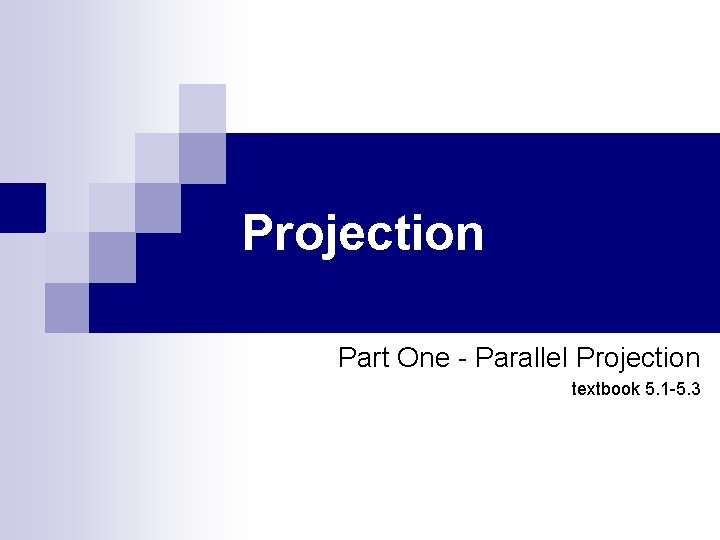Projection Part One - Parallel Projection textbook 5. 1 -5. 3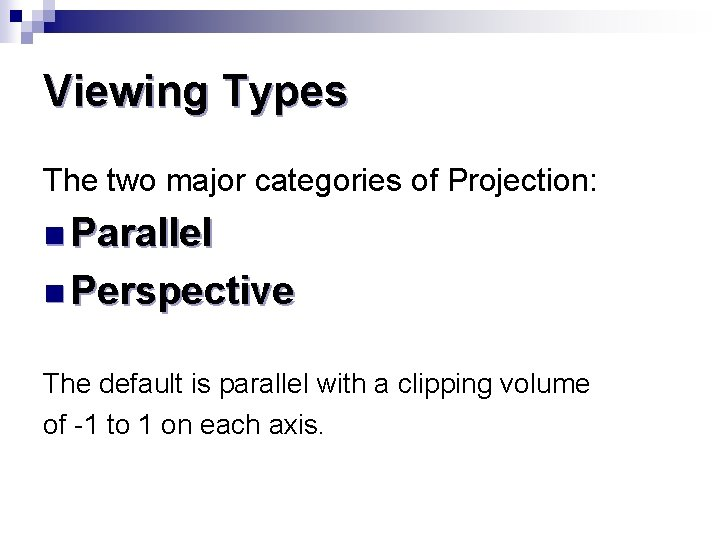Viewing Types The two major categories of Projection: n Parallel n Perspective The default is parallel with a clipping volume of -1 to 1 on each axis.Perspective www. cs. helsinki. fi http: //www. ider. herts. ac. uk/school/courseware/graphics/images/two_point_perspective. gifdocs. autodesk. com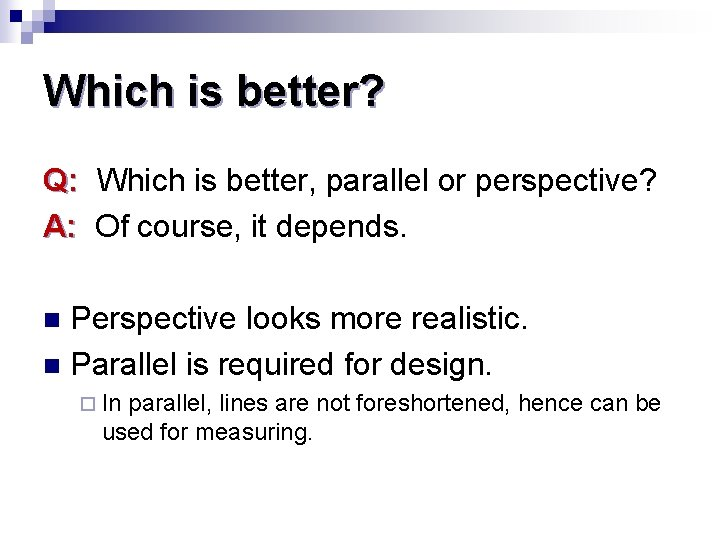Which is better? Q: Which is better, parallel or perspective? A: Of course, it depends. Perspective looks more realistic. n Parallel is required for design. n ¨ In parallel, lines are not foreshortened, hence can be used for measuring.Projection of the World onto the Projection Plane http: //local. wasp. uwa. edu. au/~pbourke/stereographics/HET 409_2003/frustum. html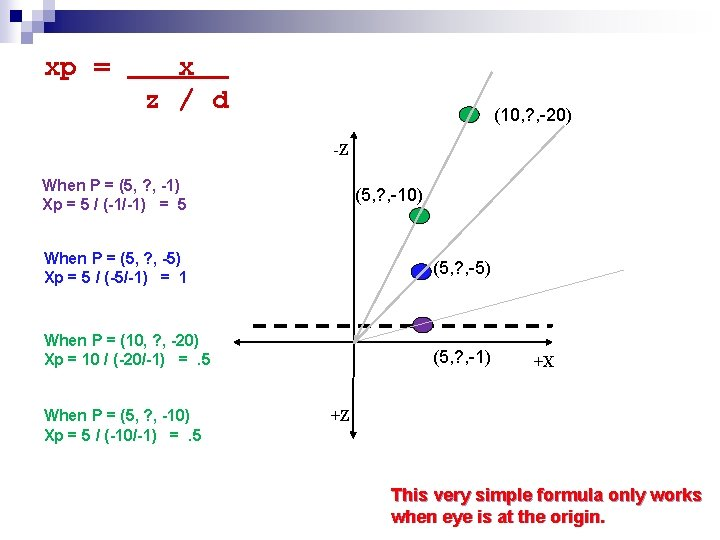xp = x z / d (10, ? , -20) -Z When P = (5, ? , -1) Xp = 5 / (-1/-1) = 5 (5, ? , -10) When P = (5, ? , -5) Xp = 5 / (-5/-1) = 1 (5, ? , -5) When P = (10, ? , -20) Xp = 10 / (-20/-1) =. 5 (5, ? , -1) When P = (5, ? , -10) Xp = 5 / (-10/-1) =. 5 +X +Z This very simple formula only works when eye is at the origin.Viewing Issues 1. We need the ability to work in units of the application. 2. We need to position the camera independently of the objects. 3. We want to be able to specify a clipping volume in units related to the application. 4. We want to be able to do either parallel or perspective projections. Angel textbook page 223Implementation n Since we do not need to work with the intermediate values, we can combine the model view matrix and the projection matrix. gl_Position = matrix * v. Position; n Or, leave them separate and have the vertex shader combine them. gl_Position = projection * modelview * v. Position; Angel textbook figure 5. 11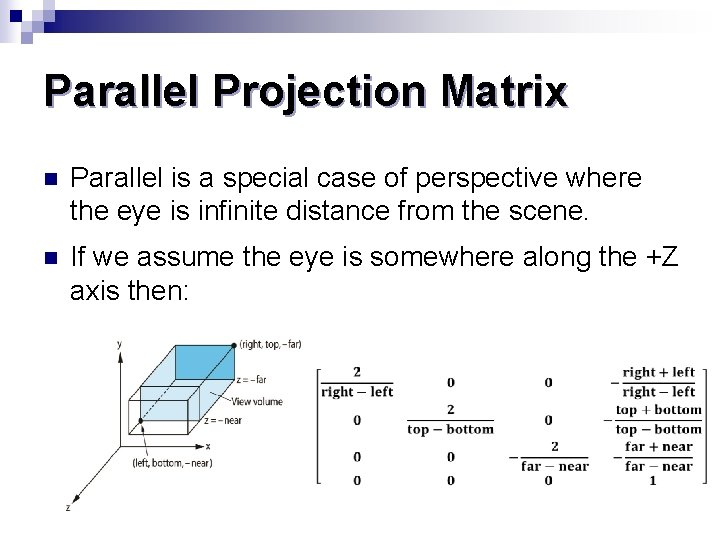Parallel Projection Matrix n Parallel is a special case of perspective where the eye is infinite distance from the scene. n If we assume the eye is somewhere along the +Z axis then: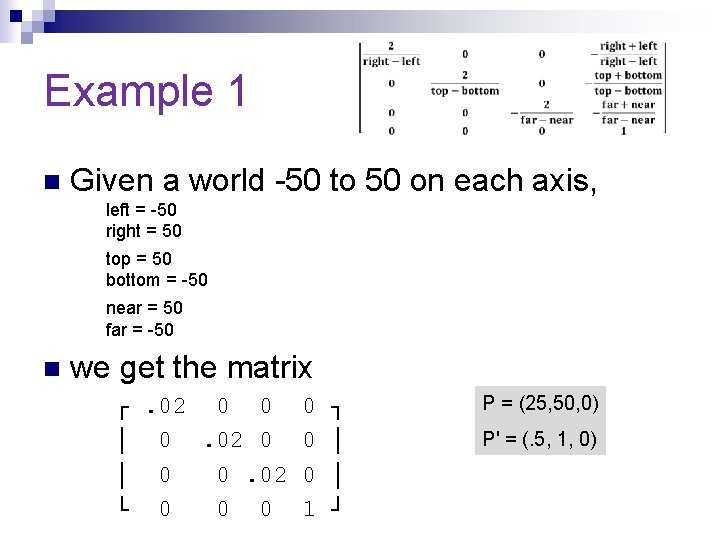Example 1 n Given a world -50 to 50 on each axis, left = -50 right = 50 top = 50 bottom = -50 near = 50 far = -50 n we get the matrix ┌. 02 0 0 0 │ 0. 02 0 0 │ 0 0. 02 0 └ 0 0 0 1 ┐ │ │ ┘ P = (25, 50, 0) P' = (. 5, 1, 0)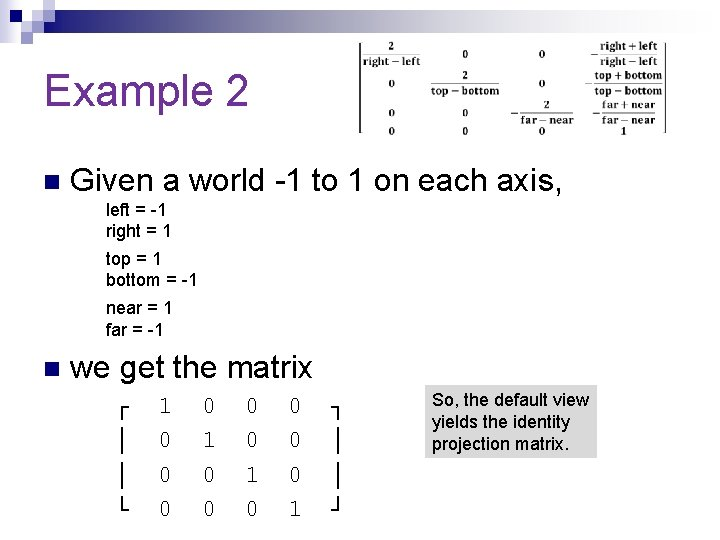Example 2 n Given a world -1 to 1 on each axis, left = -1 right = 1 top = 1 bottom = -1 near = 1 far = -1 n we get the matrix ┌ │ │ └ 1 0 0 0 0 1 ┐ │ │ ┘ So, the default view yields the identity projection matrix.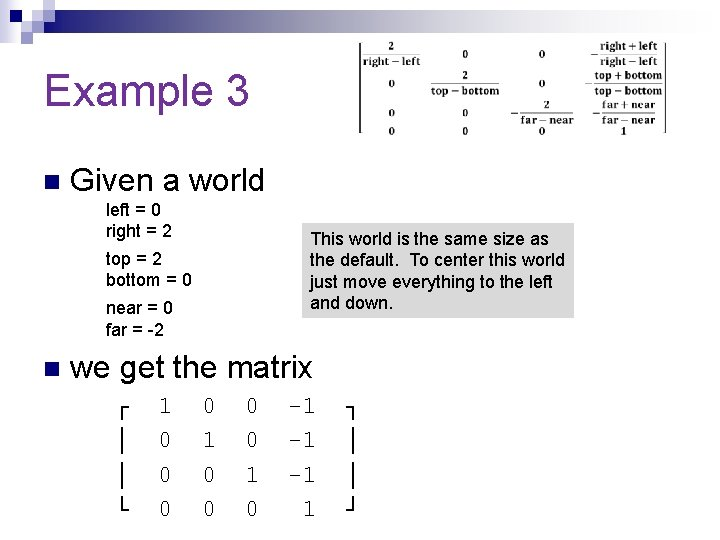Example 3 n Given a world left = 0 right = 2 This world is the same size as the default. To center this world just move everything to the left and down. top = 2 bottom = 0 near = 0 far = -2 n we get the matrix ┌ │ │ └ 1 0 0 0 0 1 0 -1 -1 -1 1 ┐ │ │ ┘Building the simple parallel matrix S = scale ( 2/(right-left), 2/(top-bottom), 2(near-far)); T = translate ( -(right+left)/2, -(top+bottom)/2, (far+near)/2); Proj. Matrix = S * T ; Angel textbook page 239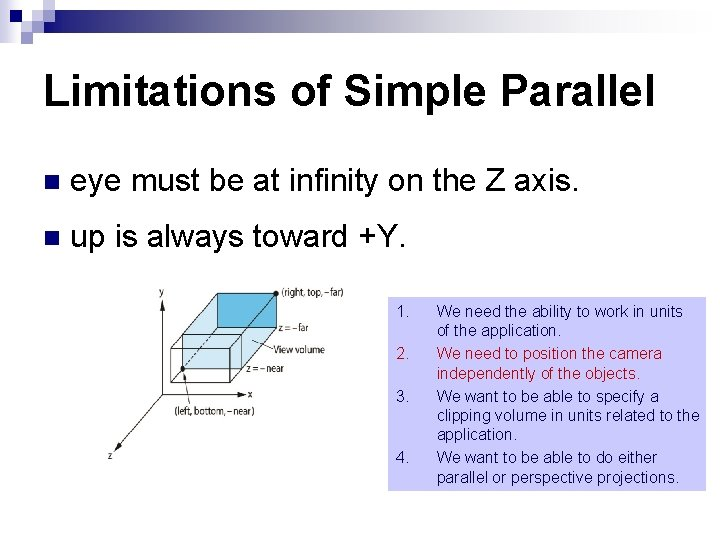Limitations of Simple Parallel n eye must be at infinity on the Z axis. n up is always toward +Y. 1. 2. 3. 4. We need the ability to work in units of the application. We need to position the camera independently of the objects. We want to be able to specify a clipping volume in units related to the application. We want to be able to do either parallel or perspective projections.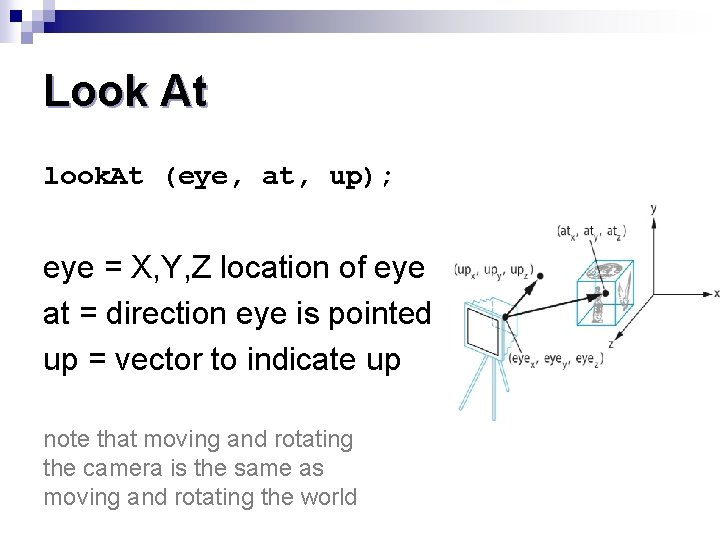Look At look. At (eye, at, up); eye = X, Y, Z location of eye at = direction eye is pointed up = vector to indicate up note that moving and rotating the camera is the same as moving and rotating the world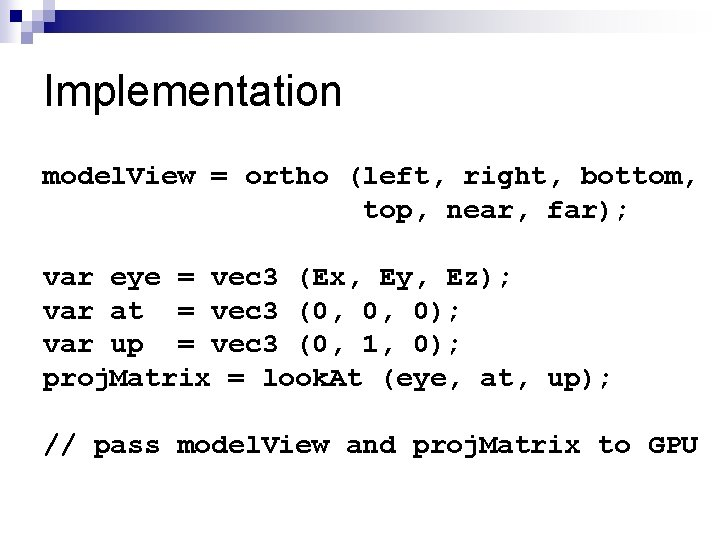Implementation model. View = ortho (left, right, bottom, top, near, far); var eye = vec 3 (Ex, Ey, Ez); var at = vec 3 (0, 0, 0); var up = vec 3 (0, 1, 0); proj. Matrix = look. At (eye, at, up); // pass model. View and proj. Matrix to GPUFrustrum Matrix A B C D E F = = = (right+left)/(right-left) (top+bottom)/(top-bottom) -(far+near)/(far-near) -2*far*near/(far-near) 2 * near/(right-left) 2 * near/(top-bottom)